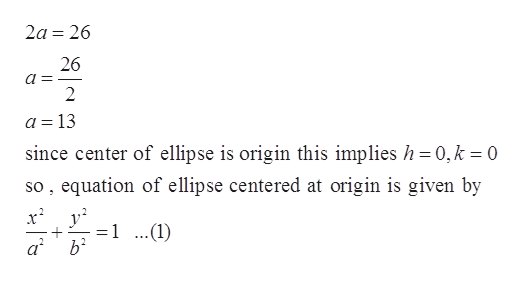# Find an equation for the ellipse that satisfies the given conditions. Length of major axis: 26, foci on x-axis, ellipse passes through the point, (square root of 13,6) centered at the origin

Question
4 views

Find an equation for the ellipse that satisfies the given conditions.
Length of major axis: 26, foci on x-axis, ellipse passes through the point, (square root of 13,6) centered at the origin

check_circle

Step 1

Given:

Length of major axis is 26 and foci on x-axis.

Step 2

Equation of ellipse is given by

Step 3

Since foci is on x-axis , leng...help_outlineImage Transcriptionclose2a 26 26 2 a =13 since center of ellipse is origin this implies h 0,k 0 so, equation of ellipse centered at origin is given by 1 ..(1) ab fullscreen

### Want to see the full answer?

See Solution

#### Want to see this answer and more?

Solutions are written by subject experts who are available 24/7. Questions are typically answered within 1 hour.*

See Solution
*Response times may vary by subject and question.
Tagged in

### Calculus